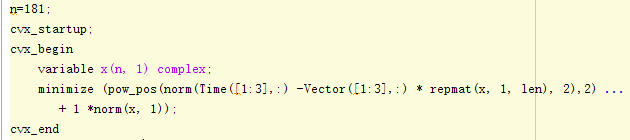# A problem about the different results from mixed matrixsI am working on the problem of the estimation of direction of arrival. In the picture，Time is the receving signal of array which is a 9x100 matrix and Vector is a 9x100 matrix representing the signal phase changing between antennas suppose to be. x is sparse representation of DOA and is a 180x1 variable means the 180 degress in space. The angle of arrival is the index of max value of s. Now i mixed 3 situations with various antenna distance with 0.5，1，2 and bulid a 9x100 Time matrix contianing three 3x100 matrixes. If i calculate these 3 matrixes seperately there results are close， for example ，the index of max value in x are 11，10，10 for a DOA of 10. But after i mix them into a 9x100 matrix the index of max value will change to 7. I’. confused about the result after mixed. Why the index is not in 10 or 11 but 7？

This is not an electrical engineering modeling forum. So I don’t think it is the right place to get assistance as to why solutions of various models come out as they do.

I just want to know if it is reasonable for a mixed matrix estimate a different result from other 3 results. Because I recognize the additional matrixes as more constrains so i think the result should be in the results from other small matrixes.

OK，thank you for your reply